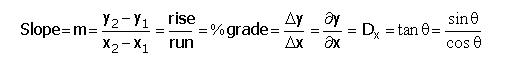# THE ULTIMATE SLOPE EQUATION

Here is the ultimate slope equation:Explanation:

• Slope = The amount of tilt of a line
• m = The symbol for slope
• (y2 - y1) / (x2 - x1) = Calculation of the slope from two points
• rise / run = Empirical definition of slope
• % grade is slope, when the percentage is converted to a decimal
• Δy / Δx = The change in y, divided by the change in x
• ∂y / ∂x = A differential is a slope
• Dx = The derivative of a function is its instantaneous slope
• The tangent of the angle of the line is its slope.
• sin θ / cos θ = The trigonometric version of the rise divided by the run.

The derivative of the slope-intercept form of a line is the slope of that line:

Dx(mx + b) = m